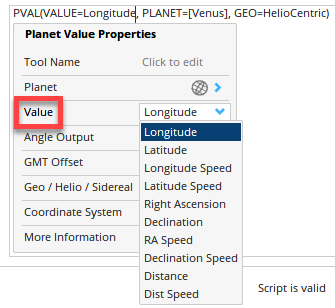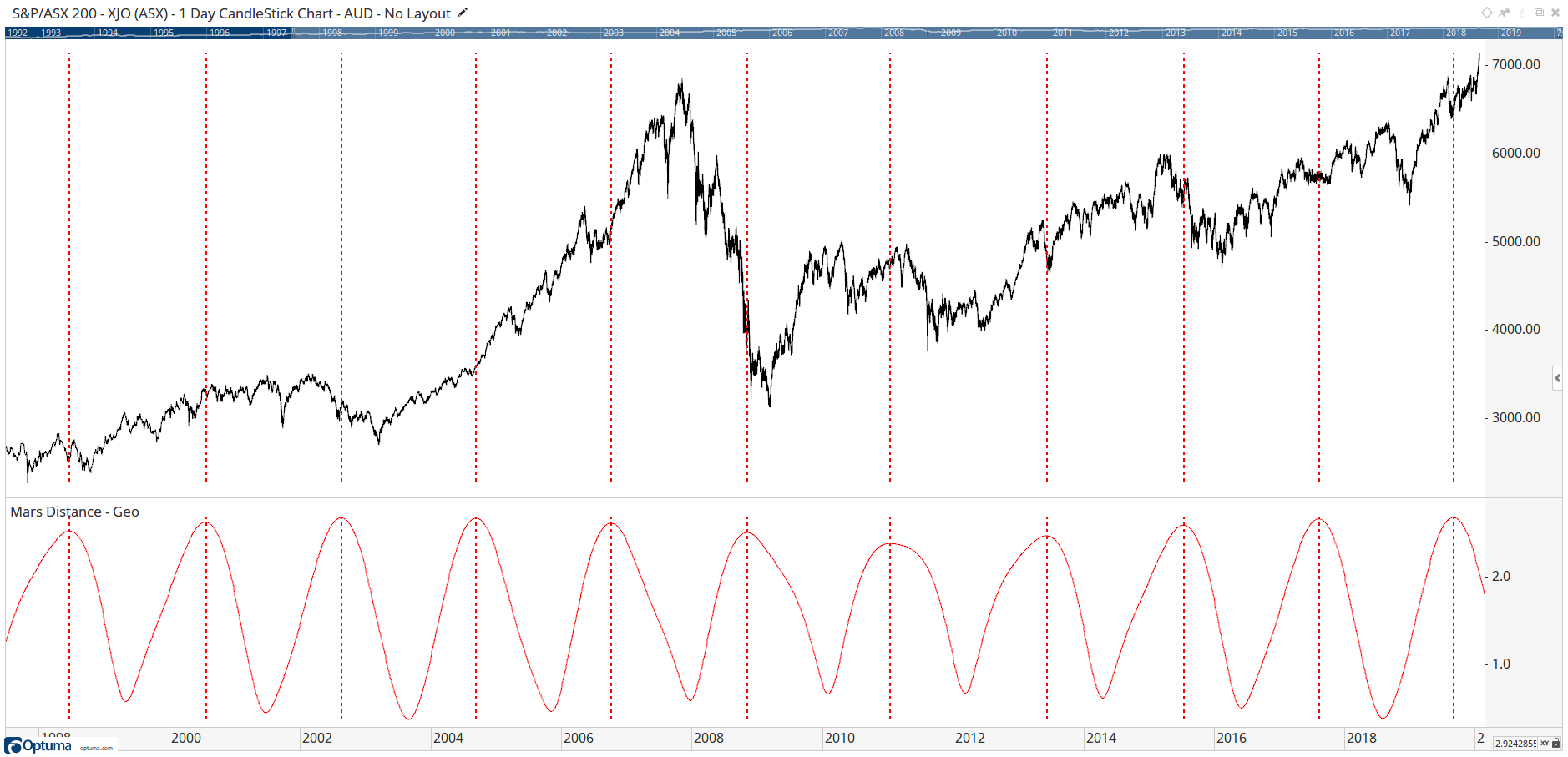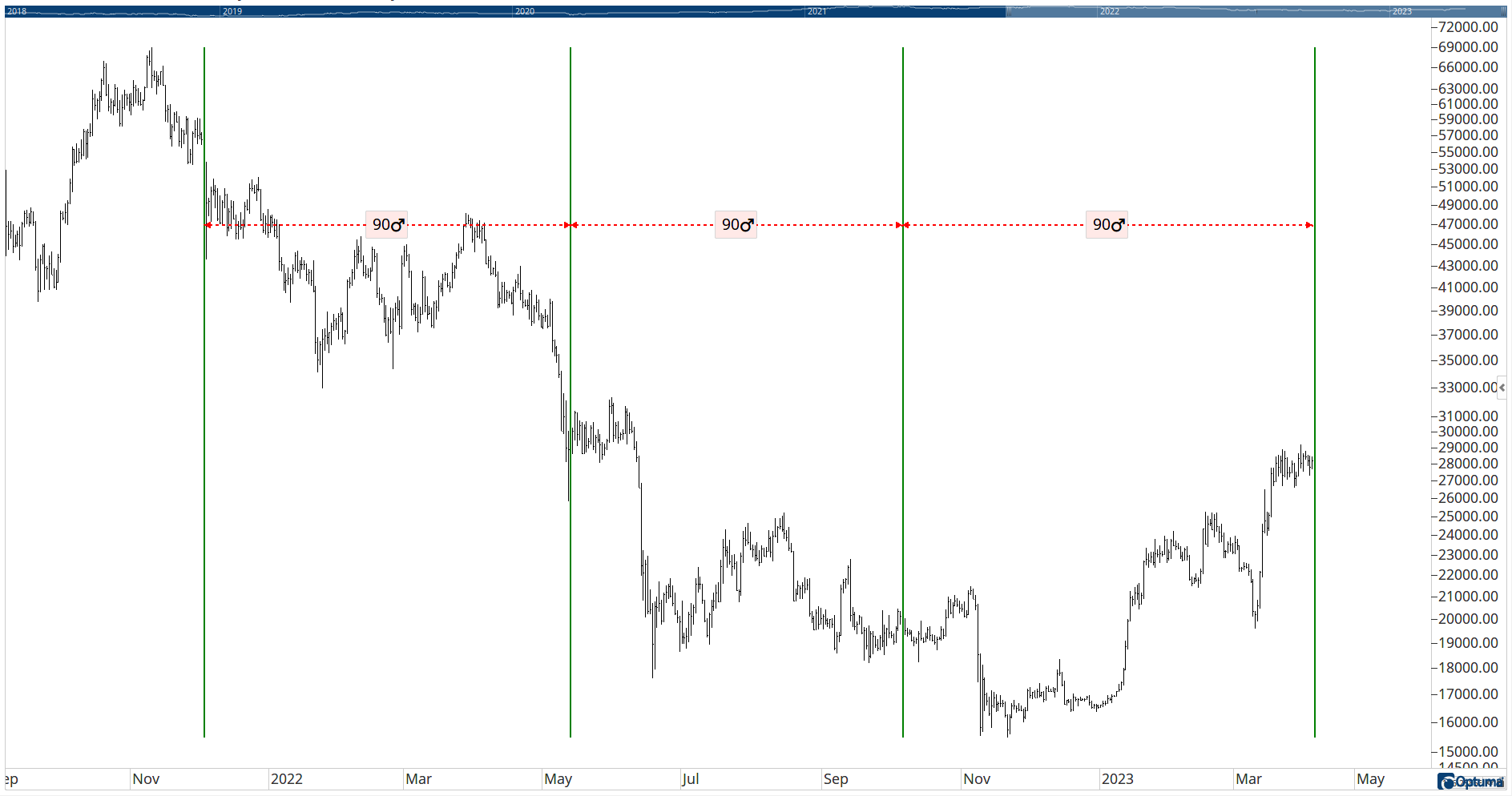# Astro Scripting Functions

Author: Optuma Team Last updated: Apr 6, 2023 11:36

For clients with the advanced astrological module it’s possible to create scripts on a number of astro tools and planetary values, such as GPLs, Planetary Aspects, and Planetary Signs. These can then be used in scans, custom tools, and backtests.

Clients with the astro module can sign in here to see the astro scripting forum to post questions and see examples:

https://forum.optuma.com/forum/astro-scripting/

See below for examples of the following functions:

### Planetary Values - PVAL()One of the most versatile astro functions is the Planetary Value PVAL(). Click on the Value field to select one of the following measures, from either a Geocentric, Heliocentric, Sidereal, or Topocentric perspective:

• Longitude
• Latitide
• Longitude Speed
• Latitude Speed
• Right Ascension
• Declination
• Right Ascension Speed
• Declination Speed
• Distance
• Distance Speed

To show the distance of Mars from Earth in a Show View:

``````PVAL(VALUE=Distance, PLANET=[Mars], GEO=GeoCentric)
``````

To show when Mars starts to move closer to Earth using Show Bar vertical lines:

``````PVAL(VALUE=Distance, PLANET=[Mars], GEO=GeoCentric) TurnsDown
``````### Planetary Aspects - PA()

To show when Mercury is conjunct the Sun:

``````PA(PLANETS1=[Sun], PLANETS2=[Mercury], ASPECTS=[Conjunction])
``````

### Gann Planetary Angles - GPA()

Used to convert planetary degrees to price. The following will scan for when price is within 3° of Venus GPL:

``````//Select Planet(s)
p1=PVAL(PLANET=[Venus]);

//Calculate Gann Planetary Angle
g1=GPA();

//Choose required aspects and orb
ASPECTS(g1, p1, ORB=3.0, ASPECTS=[Conjunction])
``````

### Planetary Angles - PAG()

Venus at 144°:

``````PAG(PLANETS1=[Venus], DEGREES=144)
``````

### Planetary Declination - PDC()

To signal when Venus is at maximum declination:

``````V1=PDC(GEO=HelioCentric, PLANETS=[Venus]) TurnsDown;
V1[-1]
``````

When Mars and Jupiter are in retrograde at the same time:

``````RL(PLANET1=[Mars]) and RL(PLANET1=[Jupiter])
``````

### Planetary Signs - PLS()

To show when Mars is in Aries:

``````PLS(PLANETS1=[Mars], SIGNS=[Aries]) == 1
``````

### Planetary Sign Changes - PLSC()

To show when Venus moves in to Leo:

``````PLSC(PLANETS=[Venus], SIGNS=[Leo]) == 1
``````

### Planetary Speed - PSP()

When Mars (Heliocentric) starts to speed up:

``````V1=PSP(GEO=HelioCentric, PLANETS=[Mars]) TurnsUp;
V1[-1]
``````

### Moon Phases - MOON()

To signal every new moon in a Show Bar or Backtest:

``````MOON(ASPECTS=[New Moon])
``````

### Eclipses - ECL()

For solar eclipses when the sun is conjunct or square Mercury:

``````ECL(ECLIPSE=Solar) and
PA(PLANETS1=[Sun], PLANETS2=[Mercury], ASPECTS=[Conjunction,Square])
``````

### Planetary Angle Difference - PDIFF()

For when Jupiter and Saturn are 120° apart:

``````PDIFF(PLANET1=[Jupiter], PLANET2=[Saturn]) Crosses 120
``````

### Time by Planetary Degrees - PDI()

To use a Show Bar to highlight each 90° movement in Mars prior to the last bar (heliocentric basis).

``````\$V = BARDATE(LAST());
V1 = PDI(LEVELS=, PLANETS=[Mars], DIRECTION=Reverse, STARTDATE=\$V, GEO=HelioCentric);
V1 == 1
``````